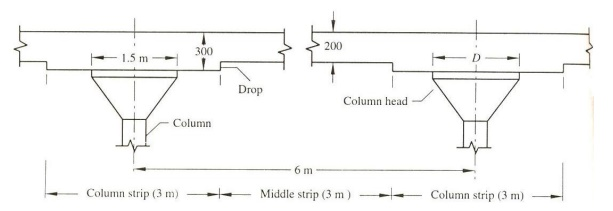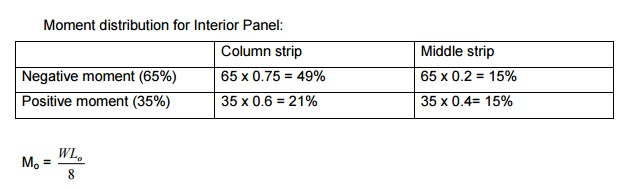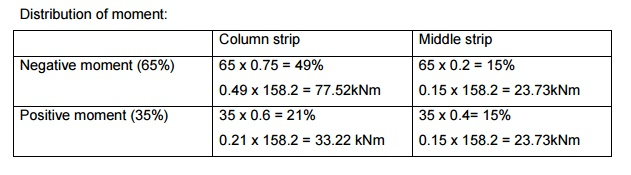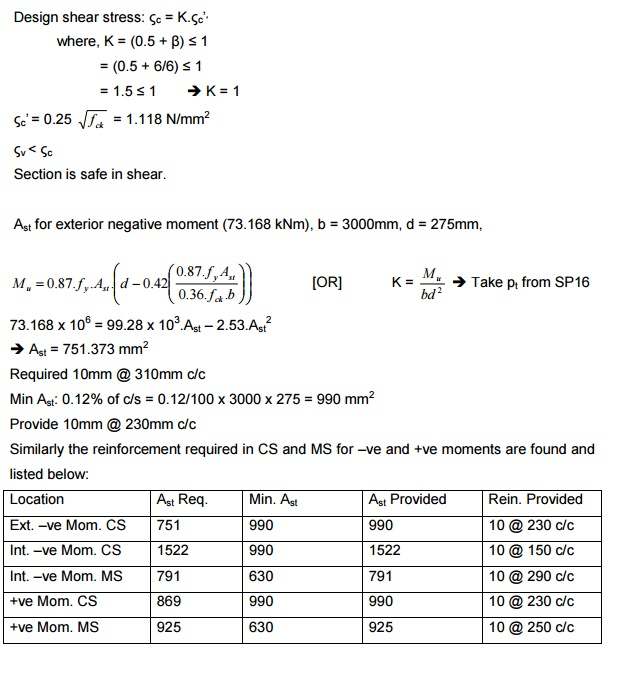# Design Of Flat Slaba) Interior panel b) Exterior panel Various components of flat slab: i) Without drop and head ii) With drop and without head iii) With drop and head

DESIGN OF FLAT SLAB

a)   Interior panel

b)   Exterior panel

Various components of flat slab:

ii)            With drop and without head

Column strip : It is the design strip having a width of l2/4, where l2 is the span transverse to l1. l2 -longer span, moment is considered along the span l1

Middle strip : It is the design strip bounded by a column strip on its opposite sides

Proportioning of flat slabs:

As per cl.31 of IS456-2000, the span by depth ratio of two way slab is applicable for flat slabs and the values can be (l/d)modified by 0.9 for flat slabs with drops.

Take l/d as 32 for HYSD bars

As per ACI -The drop thickness should not be less than 100mm or (Thickness of slab)/4. While calculating span by depth ratio, longer span is used.

The thickness of slab should not be less than 125mm.

The purpose of column drop is to reduce the shear stress and also reduce the reinforcement in the column strip.

The increase in column diameter at the head flaring of column head takes care of punching shear developed at a distance of d/2 all around the junction between the slab and column head.

Two methods of design are available for flat slabs:

1.   Direct design method

2.   Equivalent frame method

Direct design method: (Cl.31.4.1, IS456-2000)

Requirements for direct design methods are,

1.   There must be atleast three continuous spans in each direction

The panels should be rectangular with ly/lx = l2/l1 ratio < 2

3.   The columns must not offset by more than 10% of the span from either of the successive columns

4.   Successive span length in each direction must not differ by more than one third of longer span.

Design procedure:

As per Cl.31.4.2.2, IS456-2000, the total moment for a span bounded by columns laterally is Mo = Wlo/2, where Mo is the sum of positive and negative moment in each direction. W is the total design load covered on an area L2L1

W = w x L2 x Ln

This moment is distributed for the column strip and middle strip

Moment distribution for Interior Panel:Also Lo should not be less than 0.65 times of L1 [Ln > 0.65L1)

1) Design a flat slab system (interior panel) to suit the following data: Size of the floor = 20 x 30m

Column interval = 5m c/c Live load on slab = 5kN/m2

Materials used are Fe415 HYSD bars and M20 concreteProportioning of flat slab:

Assume l/d as 32, d = 5000/32 d = 156.25mm

d = 175mm (assume), D = 175 + 20 + 10/2 = 200mm

As per ACI code, the thickness of drop > 100mm and > (Thickness of slab)/4 Therefore, 100mm or 200/4=50mm

Provide a column drop of 100mm

Overall depth of slab at drop = 200 + 100 = 300mm Length of the drop > L/3 = 5/3 = 1.67m

Provide length of drop as 2.5m. For the panel, 1.25m is the contribution of drop. Column head = L/4 = 5/4 = 1.25m

L1 = L2 = 5m

Ln = L2 -D = 5 -1.25 = 3.75m

As per code, Mo = WLo/8Self weight of slab = 25 x 0.25 = 6.25 kN/m2

Floor finish = 0.75 kN/m2

Total = 12 kN/m2

Factored load = 1.5 x 12 = 18 kN/m2

W = wu x L2 x Ln = 18 x 5 x 3.75 = 337.5 kN

Total moment on slab panel = (337.5 x 3.75)/8 = 158.203 kNm

Distribution of moment:Column strip:

Mu = 0.138.fck.b.d2

b = 2.5m

77.5 x 106 = 0.138 x 20 x 2.5 x 1000 x d2

d = 105.98mm ~ 106mm < 275mm Middle strip:

Mu = 0.138.fck.b.d2       b = 2.5m

23.73 x 106 = 0.138 x 20 x 2.5 x 1000 x d2

d = 58.68mm ~ 59mm < 175mmCheck for punching shear:

The slab is checked for punching shear at a distance of d/2 all around the face of the column head. The load on the slab panel excluding the circular area of diameter (D + d) is the punching shear force.

= 18 x 5 x 5 -(?(D 2/4)+x wd)n

= 417.12 kN

Shear force along the perimeter of the circular area = ShearForce / p(D +d )   = 87.06 kN

Nominal shear stress: (b = 1m)Required 10mm @ 240mm c/c

Min Ast: 0.12% of c/s = 0.12/100 x 1000 x 275 = 825 mm2

Provide 10mm @ 230mm c/c

Positive moment = 33.2 kNm

Ast = 337.876 mm2

Provide 8mm @ 370mm c/c

Min. steel: Provide 10mm @ 230mm c/c

Middle strip: (b = 2.5m),   (d = 175mm)

Negative and positive moment: 23.7 kNm Ast = 382.6 mm2

Ast min. = (0.12/100 x 1000 x 2500 x 175) = 525 mm2

Provide 8mm @ 230mm c/c.The distribution of interior negative moment for column strip and middle strip is in the ratio 3:1 (0.75 : 0.25)

The exterior negative moment is fully taken by the column strip. The distribution of positive moment in column strip and middle strip is in the ratio 1.5 : 1 (0.6 : 0.4).

Design an exterior panel of a flat slab floor system of size 24m x 24m, divided into panels 6m x 6m size. The live load on the slab is 5 kN/m2 and the columns at top and bottom are at diameter 400mm. Height of each storey is 3m. Use M20 concrete and Fe415 steel.

l/d = 32  d = 6000/32 = 187.5 mm

Length          of   drop   ?   3m

Length of drop = Column strip = 3m

Assume effective depth, d = 175mm, D = 200mm As per ACI, Assume a drop of 100mm

Depth of slab at the drop is 300mm Diameter of column head = l/4 = 6/4 = 1.5m

Self weight of slab = (0.2 + 0.3)/2 x 25 = 0.25 x 25 = 6.25 kN/m2

Floor finish = 0.75 kN/m2

Total = 12 kN/m2

Factored load = 1.5 x 12 = 18 kN/m2Study Material, Lecturing Notes, Assignment, Reference, Wiki description explanation, brief detail
Civil - Design Of Reinforced Concrete And Brick Masonry Structures- Design Of Flat Slab : Design Of Flat Slab |

Related Topics